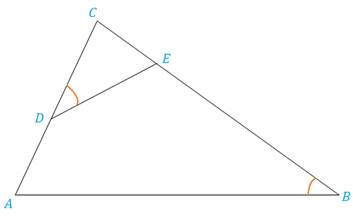Chapter 5.3, Problem 30EElementary Geometry For College St...

7th Edition
Alexander + 2 others
ISBN: 9781337614085

Solutions

Chapter
SectionElementary Geometry For College St...

7th Edition
Alexander + 2 others
ISBN: 9781337614085
Textbook Problem

Δ C D E ∼ Δ C B A with ∠ C D E ≅ ∠ B . If C D = 10 , C A = 16 and E B = 12 , find CE.Exercises 29, 30

To determine

To find:

The side CE by using the given information.

Explanation

Definition:

CSSTP:

Corresponding sides of similar triangles are proportional.

Description:

Given that ΔCDEΔCBA with CDEB. If CD=10,CA=16 and EB=12.

The given figure is shown below.

Figure 1

From the given figure, it is observed that there are two triangles CDE and CBA and which is given that two triangles are similar.

Now draw the two triangles separately as shown below.

Figure 2

Figure 3

Since ΔCDEΔCBA and from the definition of CSSTP, corresponding sides of similar triangles are proportional.

That is, CDCB=CECA=DEBA.

It is given that CD=10,CA=16 and EB=12.

Find the side CE.

Consider the first two pairs of proportions CDCB=CECA in order to find CE.

From Figure 1, it is observed that CB=CE+EB

Still sussing out bartleby?

Check out a sample textbook solution.

See a sample solution

The Solution to Your Study Problems

Bartleby provides explanations to thousands of textbook problems written by our experts, many with advanced degrees!

Get Started

Find the x- and y-intercepts of the graph of y=x22x3.

Calculus: An Applied Approach (MindTap Course List)

Solve the equations in Exercises 112 for x (mentally, if possible). x3=1

Finite Mathematics and Applied Calculus (MindTap Course List)

For , f(x) = 0 2 3 f(3) does not exist

Study Guide for Stewart's Multivariable Calculus, 8th